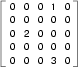# 12.5. Sparse Matrices¶

A matrix is a two dimensional collection, typically thought of as having rows and columns of data. One of the easiest ways to create a matrix is to use a list of lists. For example, consider the matrix shown below.We can represent this collection as five rows, each row having five columns. Using a list of lists representation, we will have a list of five items, each of which is a list of five items. The outer items represent the rows and the items in the nested lists represent the data in each column.

```matrix = [[0, 0, 0, 1, 0],
[0, 0, 0, 0, 0],
[0, 2, 0, 0, 0],
[0, 0, 0, 0, 0],
[0, 0, 0, 3, 0]]
```

One thing that you might note about this example matrix is that there are many items that are zero. In fact, only three of the data values are nonzero. This type of matrix has a special name. It is called a sparse matrix.

Since there is really no need to store all of the zeros, the list of lists representation is considered to be inefficient. An alternative representation is to use a dictionary. For the keys, we can use tuples that contain the row and column numbers. Here is the dictionary representation of the same matrix.

```matrix = {(0, 3): 1, (2, 1): 2, (4, 3): 3}
```

We only need three key-value pairs, one for each nonzero element of the matrix. Each key is a tuple, and each value is an integer.

To access an element of the matrix, we could use the `[]` operator:

```matrix[(0, 3)]
```

Notice that the syntax for the dictionary representation is not the same as the syntax for the nested list representation. Instead of two integer indices, we use one index, which is a tuple of integers.

There is one problem. If we specify an element that is zero, we get an error, because there is no entry in the dictionary with that key. The alternative version of the `get` method solves this problem. The first argument will be the key. The second argument is the value `get` should return if the key is not in the dictionary (which would be 0 since it is sparse).

Lab

• Counting Letters In this guided lab exercise we will work through a problem solving exercise that will use dictionaries to generalize the solution to counting the occurrences of all letters in a string.

Lab